# Texas Go Math Grade 4 Lesson 13.2 Answer Key Classify Triangles

Refer to our Texas Go Math Grade 4 Answer Key Pdf to score good marks in the exams. Test yourself by practicing the problems from Texas Go Math Grade 4 Lesson 13.2 Answer Key Classify Triangles.

## Texas Go Math Grade 4 Lesson 13.2 Answer Key Classify Triangles

Essential Question

How can you classify triangles by the size of their angles?
Yes, Triangles can also be classified by their angles.

Explanation:
In an acute angle triangle, all three angles are acute (i.e less than 90 degrees). And a right triangle contains one right angle and two acute angles. Also, an obtuse triangle contains one obtuse angle (i.e greater than 90 degrees) and two acute angles.

Unlock the Problem

A triangle is a polygon with three sides and three angles. You can name a triangle by the vertices of its angles.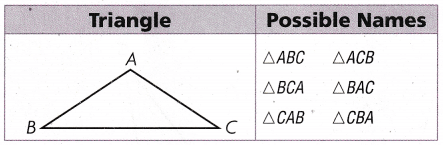An angle of a triangle can be right, acute, or obtuse.

When you see “∆ ABC,” say “triangle ABC.”

Activity 1 Identify right, acute, and obtuse angles in triangles.

Materials

• color pencils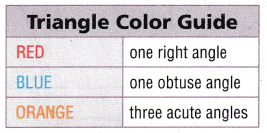Use the Triangle color Guide to color the triangles below.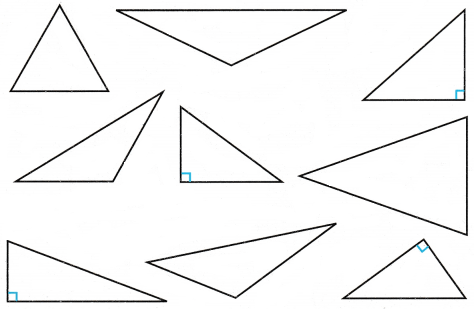Math Talk

Mathematical Processes
Can a triangle have more one obtuse Explain.
Triangle cannot have more than one obtuse angle because there are three sides and if you add three sides it is equal to 180 degrees and an obtuse angle is greater than 90 degree and the other two has to be less than 90 degrees.

Try This!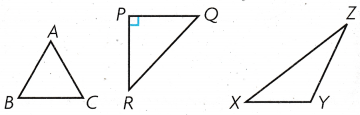a. Name the triangle with one right angle. ____________
∠QPR

Explanation:
The triangle with one right angle ∠PQR.

b. Name the triangle with one obtuse angle. ____________
∠XYZ

Explanation:
The triangle with one right angle ∠XYZ

c. Name the triangle with three acute angles. ____________
∠ABC

Explanation:
The triangle with one right angle ∠ABC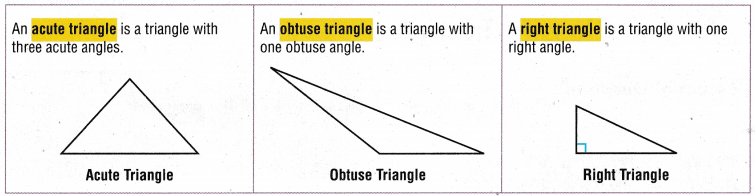Activity 2 Use a Venn diagram to classify triangles.

Write the names of the triangles in the Venn diagram.Acute triangles
∠SRT, ∠LMN, ∠ACB
Right Triangles
∠BCD, ∠PQR
Obtuse Triangles
∠WVX, ∠EFD, ∠HIG

Math Talk

Mathematical Processes
Explain why the three circles in this Venn diagram do not overlap.

Share and Show

Question 1.
Name the triangle. Tell whether each angle is acute, right, or obtuse.

A name for the triangle is ____________ .∠F is _____________ .
∠G is _____________ .
∠H is _____________ .
Right angled traingle.

Explanation:
∠F is an acute-angled triangle
∠G is a right-angled triangle
∠H is an obtuse-angled triangle.

Classify each triangle. Write acute, right, or obtuse.

Question 2.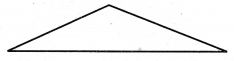Right-angled triangle

Question 3.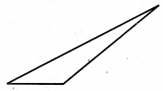Obtuse triangle

Question 4.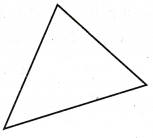Acute triangle.

Problem Solving

Use the Venn diagram for 5-6.Question 5.H.O.T. Multi-Step Which triangles do NOT have an obtuse angle? Explain.
∆ABC and ∆GHP

Explanation:
The triangles that do NOT have an obtuse angle are right triangles i.e ∆ABC and ∆GHP

Question 6.
H.O.T. Communicate How many triangles have at least two acute angles? Explain.
Right angle triangles and obtuse triangles have at least two acute angles.

Question 7.
What type of triangle is ∆ABC?(A) right
(B) acute
(C) obtuse
(D) straight
Acute

Explanation:
The type of triangle is ∆ABC is an acute angle triangle.

Question 8.
Bobby built a sandbox in the shape of the triangle below. Which type of triangle did he use to build the sandbox?(A) obtuse
(B) right
(C) equilateral
(D) acute
Right

Explanation:
The type of triangle he used to build the sandbox is a right-angled triangle.

Question 9.
Multi-Step Laura drew and labeled the triangles below. She wants to color the obtuse triangle red. Which triangle should Laura color red?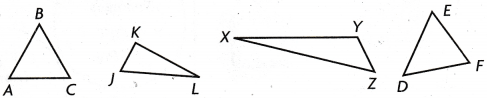(A) ∆ABC
(B) ∆DEF
(C) ∆XYZ
(D) ∆JKL
∆XYZ

Explanation:
The triangle should Laura’s color red is ∆XYZ.

TEXAS Test Prep

Question 10.
How many acute angles are in an obtuse triangle?
(A) 0
(B) 1
(C) 2
(D) 3
2

Explanation:
There are 2 acute angles in an obtuse angle triangle.

### Texas Go Math Grade 4 Lesson 13.2 Homework and Practice Answer Key

Question 1.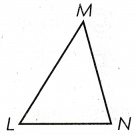Acute

Question 2.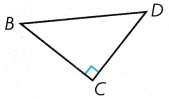Right.

Question 3.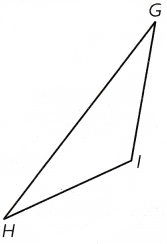Obtuse

Question 4.Right

Question 5.Obtuse

Question 6.Acute

Question 7.
Name the triangle. Tell whether each angle is acute, right or obtuse.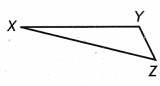A name for the triangle is ___________________________
∠X is _______________. ∠Y is _______________. ∠Z is _______________.
Obtuse
∠X is acute.
∠Y is obtuse.
∠Z is acute.

Explanation:
A name for the triangle is Obtuse.

Problem Solving

Question 8.
Mark folded a flag into a triangle and built a frame for it in the shape of the triangle below. Which type of triangle did he use to build the frame?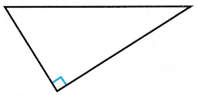Right.

Explanation:
The type of triangle he used to build the frame is a Right-angled triangle.

Question 9.
Cassie added a flower garden to the corner of her yard in the shape of the triangle below. Which type of triangle did she use to add to the garden?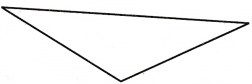Obtuse

Explanation:
The type of triangle she used to add to the garden is an obtuse-angled triangle.

Lesson Check

Question 10.
What type of triangle is ∆ DEF?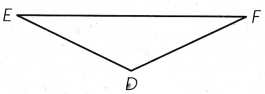(A) acute
(B) right
(C) obtuse
(D) equilateral
Obtuse

Explanation:
The triangle ∆ DEF is an obtuse angle triangle.

Question 11.
What type of triangle is ∆ JKL?(A) acute
(B) obtuse
(C) straight
(D) right
Acute

Explanation:
The triangle ∆ JKL is an acute triangle that has less than 90°

Question 12.
How many acute angles are in a right triangle?
(A) 0
(B) 1
(C) 2
(D) 3

Question 13.
Which triangle has one right angle?(A) ∆VWX
(B) ∆TUV
(C) ∆RST
(D) ∆BCD
∆BCD

Explanation:
The ∆BCD has one right angle.

Question 14.
Multi-Step Which triangle has both acute and obtuse angles?(A) ∆DEF
(B) ∆ABC
(C) ∆PQR
(D) ∆LMN
∆ABC

Explanation:
The triangle ∆ABC has both acute and obtuse angles.

Question 15.
Multi-Step Which triangle has one right angle and no obtuse angles?(A) ∆ ABC
(B) ∆ GHI
(C) ∆ PQR
(D) ∆ LMN